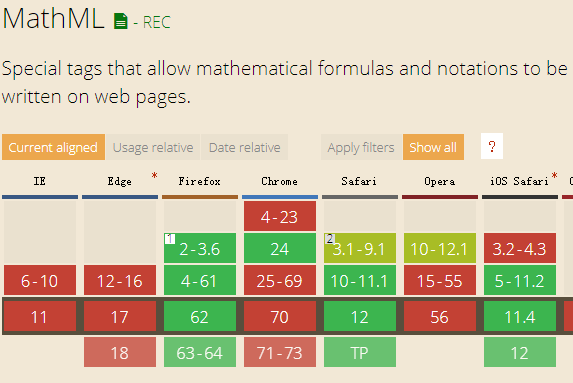# HTML5 数学标记语言

## 1. 发展史

### 1.1 版本

• 1.01 版于 1999 年 7 月公布
• 2.0 版于 2001 年 2 月出现。
• 万维网联盟在 2003 年 10 月发布了MathML 2.0 的第二版
• 2010 年 10 月发布了 MathML 3.0。

### 1.2 作用

MathML 包含两个子语言：Presentation MathML 和 Content MathML。Presentation MathML 主要负责描述数学表达式的布局。Content MathML 主要负责标记表达式的某些含义或数学结构。MathML 的这一方面受到 OpenMath 语言的很大影响，在 MathML3 中，与 OpenMath 更为贴近。为什么需要使用 MathML 呢？

## 2.语法简介

``````<math xmlns = "http://www.w3.org/1998/Math/MathML">
<mrow>
<mrow>
<mi>x</mi>
<mo>+</mo>
<mi>y</mi>
</mrow>
<mo>=</mo>
<mn>10</mn>
</mrow>
</math>
``````

### 2.1 mrow

mrow 标签用于对表达式进行分组，至少由一个到多个运算符组成，此元素呈水平展示。良好的分组对数学表达式结构展示起到一定的改善效果。

### 2.2 mi

mi 标签表示运算表达式中的变量，例如：

``````<math xmlns = "http://www.w3.org/1998/Math/MathML">
<mrow>
<mi>a</mi>  <!--表示变量-->
<mo>+</mo>
<mi>b</mi>  <!--表示变量-->
</mrow>
</math>
``````

### 2.3 mo

mo 标签用于表示运算符，例如：

``````<math xmlns = "http://www.w3.org/1998/Math/MathML">
<mrow>
<mi>a</mi>
<mo>-</mo><!--减法运算符-->
<mi>b</mi>
</mrow>
</math>
``````

### 2.4 mn

mn 标签用于显示表达式中的数字。

``````<math xmlns = "http://www.w3.org/1998/Math/MathML">
<mrow>
<mn>5</mn><!--表示数字-->
<mo>+</mo>
<mn>1</mn>
</mrow>
</math>
``````

### 2.5 mscarries

``````<math>
<mscarries> expression <mscarry> <none/> </mscarry> </mscarries>
</math>
``````

### 2.6 menclose

• longdiv - 精算；
• phasorangle - 激进；
• updiagonalstrike - 盒子；
• downdiagonalstrike - 圆盒；
• verticalstrike - 圆；
• horizontalstrike - 左；
• northeastarrow - 右；
• text - 底部；

### 2.7 mfenced

• open 指定开始分隔符，默认是 “（” ;
• close 指定结束分隔符，默认是 “）”;
• separators 指定零个或多个分隔符序列，默认是 “,”

``````<math xmlns = "http://www.w3.org/1998/Math/MathML">
<mrow>
<mo>(</mo>
<mn>1</mn>
<mo>)</mo>
</mrow>
</math><!--这是不适用mfenced的方式-->

<math xmlns = "http://www.w3.org/1998/Math/MathML">
<mfenced>
<mn>1</mn>
</mfenced>
</math><!--使用mfenced快捷方式-->
``````

### 2.8 mfrac

（请使用 Firefox 运行下面代码）

``````<math xmlns = "http://www.w3.org/1998/Math/MathML">
<mfrac>
<mi>y</mi>
<mn>10</mn>
</mfrac>
</math>
``````

### 2.9 mlongdiv

（请使用 Firefox 运行下面代码）

``````<mlongdiv> divisor dividend result expression </mlongdiv>
``````

### 2.10 mtable

（请使用 Firefox 运行下面代码）

``````<math xmlns = "http://www.w3.org/1998/Math/MathML">
<mtable>
<mtr>
<mtd><mn>1</mn></mtd>
<mtd><mn>0</mn></mtd>
<mtd><mn>0</mn></mtd>
</mtr>
<mtr>
<mtd><mn>0</mn></mtd>
<mtd><mn>1</mn></mtd>
<mtd><mn>0</mn></mtd>
</mtr>
</mtable>
</math>
``````

### 2.11 msgroup

（请使用 Firefox 运行下面代码）

``````<math xmlns = "http://www.w3.org/1998/Math/MathML">
<mstack>
<msgroup>
<mn>123</mn>
<msrow>
<mo>&#xD7;</mo>
<mn>321</mn>
</msrow>
</msgroup>
<msline/>
</mstack>
</math>
``````

### 2.12 mover

（请使用 Firefox 运行下面代码）

``````<math xmlns = "http://www.w3.org/1998/Math/MathML">
<mover accent = "true">
<mrow>
<mi> 1 </mi>
<mo> + </mo>
<mi> 2 </mi>
<mo> + </mo>
<mi> 3 </mi>
</mrow>
<mo>&#x23DE;</mo>
</mover>
</math>
``````

（请使用 Firefox 运行下面代码）

``````<math xmlns = "http://www.w3.org/1998/Math/MathML">
<mrow>
<mi>x</mi>
<mpadded lspace = "0.2em" voffset = "0.3ex">
<mi>y</mi>
<mi>z</mi>
</mrow>
</math>
``````

### 2.14 mphantom

（请使用 Firefox 运行下面代码）

``````<math xmlns = "http://www.w3.org/1998/Math/MathML">
<mfrac>
<mrow>
<mi> x </mi>
<mo> + </mo>
<mi> y </mi>
<mo> + </mo>
<mi> z </mi>
</mrow>

<mrow>
<mi> x </mi>
<mphantom>
<mo> + </mo>
</mphantom>

<mphantom>
<mi> y </mi>
</mphantom>
<mo> + </mo>
<mi> z </mi>
</mrow>
</mfrac>
</math>
``````

### 2.15 msqrt

（请使用 Firefox 运行下面代码）

``````<math xmlns = "http://www.w3.org/1998/Math/MathML">
<msqrt>
<mn>256</mn>
</msqrt>
</math>
``````

### 2.16 msub

（请使用 Firefox 运行下面代码）

``````<math xmlns = "http://www.w3.org/1998/Math/MathML">
<msub>
<mi>y</mi>
<mn>10</mn>
</msub>
</math>
``````

### 2.17 msubsup

（请使用 Firefox 运行下面代码）

``````<math xmlns = "http://www.w3.org/1998/Math/MathML">
<mrow>
<msubsup>
<mo> &#x222B;</mo>
<mn> 0 </mn>
<mn> 1 </mn>
</msubsup>

<mrow>
<msup>
<mi> e</mi>
<mi> x </mi>
</msup>
<mo> &#x2062;</mo>

<mrow>
<mi> d</mi>
<mi> x </mi>
</mrow>
</mrow>
</mrow>
</math>
``````

### 2.18 msup

（请使用 Firefox 运行下面代码）

``````<math xmlns = "http://www.w3.org/1998/Math/MathML">
<msup>
<mi>y</mi>
<mn>2</mn>
</msup>
</math>
``````

### 2.19 munder

（请使用 Firefox 运行下面代码）

``````<math xmlns = "http://www.w3.org/1998/Math/MathML">
<munder accent="true">
<mrow>
<mi>a </mi>
<mo> + </mo>
<mi> b </mi>
<mo> + </mo>
<mi> c </mi>
</mrow>
<mo>&#x23F;</mo>
</munder>
</math>
``````

### 2.20 munderover

（请使用 Firefox 运行下面代码）

``````<math xmlns = "http://www.w3.org/1998/Math/MathML">
<mrow>
<munderover>
<mo> &#x222B;</mo>
<mn> 0 </mn>
<mi> &#x221E;</mi>
</munderover>
</mrow>
</math>
``````

## 3.兼容性## 4.第三方工具

### 4.2 第三方库

（下面例子可以使用 chorme 浏览器试试了，平方根也是可以显示出来的）

``````<script src="//fred-wang.github.io/mathml.css/mspace.js"></script>
<math xmlns = "http://www.w3.org/1998/Math/MathML">
<msqrt>
<mn>256</mn>
</msqrt>
</math>
``````